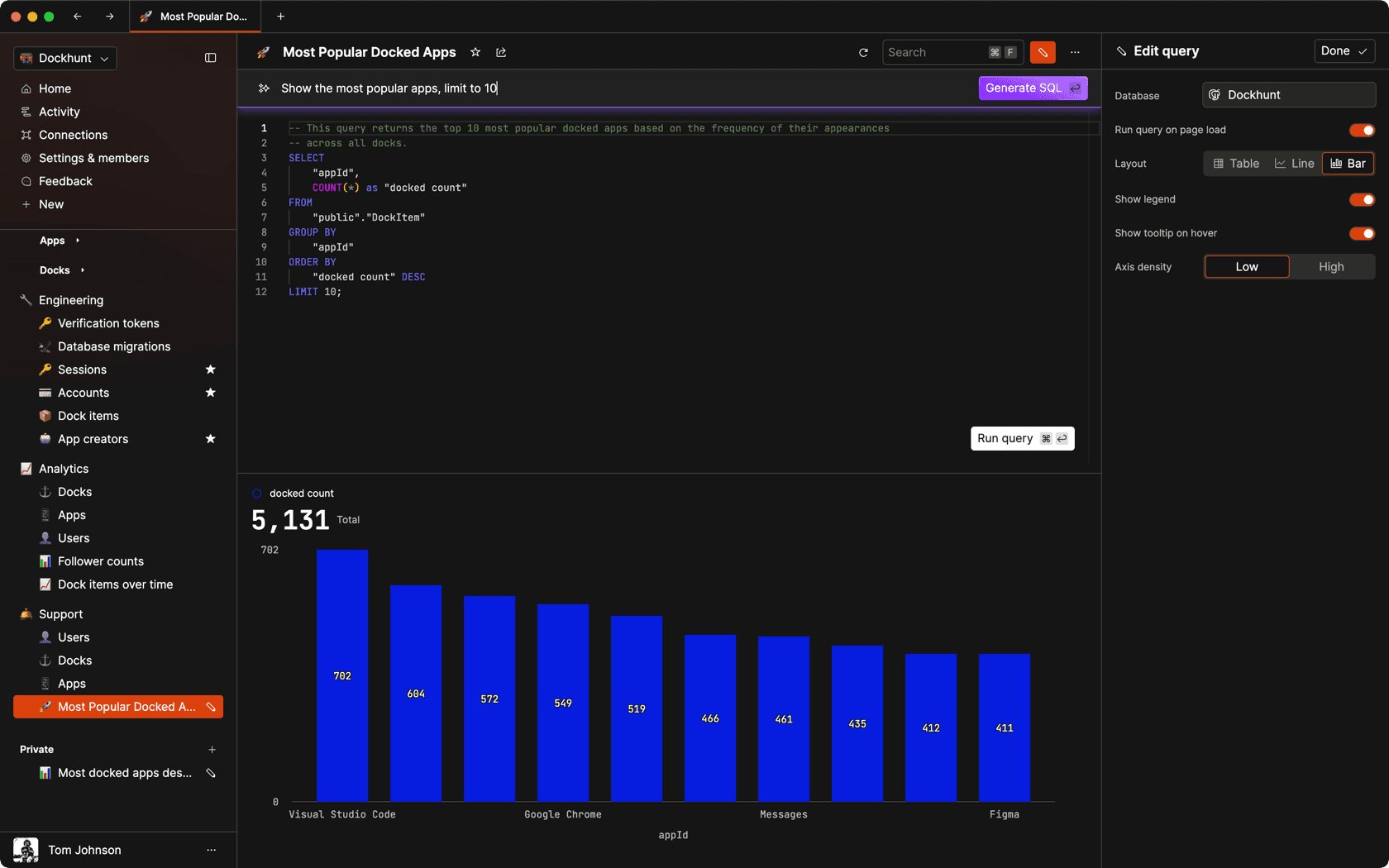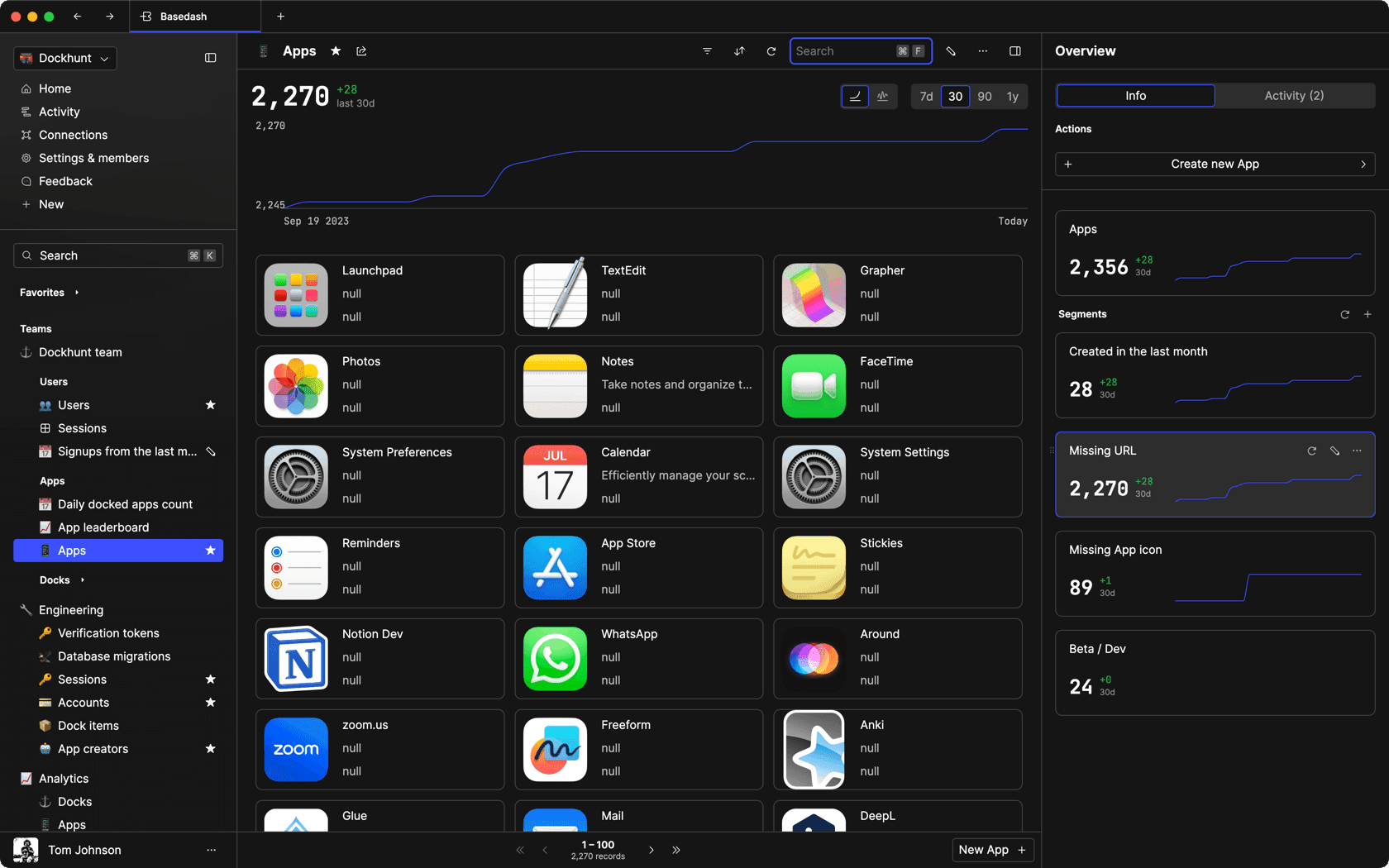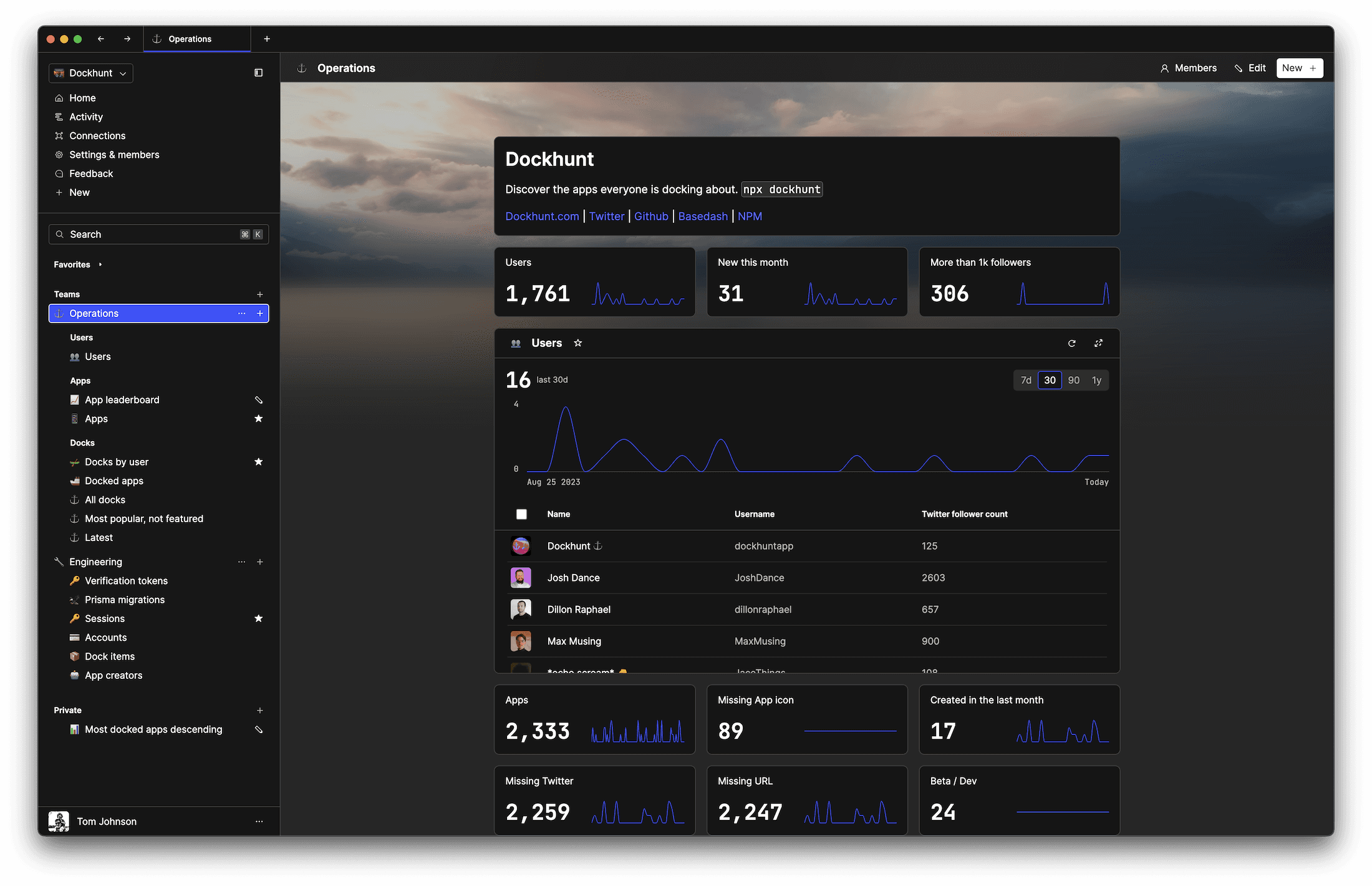# How to do integer division in JavaScriptself.__wrap_n=self.__wrap_n||(self.CSS&&CSS.supports("text-wrap","balance")?1:2);self.__wrap_b=(e,r,t)=>{t=t||document.querySelector(`[data-br="\${e}"]`);let n=t.parentElement,a=s=>t.style.maxWidth=s+"px";t.style.maxWidth="";let o,i=n.clientWidth,l=n.clientHeight,c=i/2-.25,f=i+.5;if(i){for(a(c),c=Math.max(t.scrollWidth,c);c+1<f;)o=Math.round((c+f)/2),a(o),n.clientHeight===l?f=o:c=o;a(f*r+i*(1-r))}t.__wrap_o||typeof ResizeObserver<"u"&&(t.__wrap_o=new ResizeObserver(()=>{self.__wrap_b(0,+t.dataset.brr,t)})).observe(n)};self.__wrap_n!=1&&self.__wrap_b(":Rj0pm:",1)

November 6, 2023

Unlike other programming languages, JavaScript doesn’t have a built-in operator for integer division. But you can get around this by using a combination of the division and floor functions or bitwise operators. We’ll cover how to do all this in the follow guide.

## Understanding division and the modulus operator

To start with integer division in js, you need to understand the standard division (`/`) operator and the modulus operator (`%`). The division operator returns a floating-point result, while the modulus operator returns the remainder of a division.

```let result = 10 / 3; // result is 3.333...
let remainder = 10 % 3; // remainder is 1```

## Using `Math.floor()` to achieve integer division

One way to perform integer division is to use the `Math.floor()` function, which rounds down to the nearest integer:

```let dividend = 10;
let divisor = 3;
let quotient = Math.floor(dividend / divisor); // quotient is 3```

## Using `Math.trunc()` for positive dividends

For positive numbers, `Math.trunc()` can be used as it simply truncates the fractional part, giving the integer quotient:

`let quotient = Math.trunc(10 / 3); // quotient is 3`

## Bitwise OR for fast integer division

Bitwise operators in js can also perform integer division quickly for 32-bit integers by forcing JavaScript to treat operands as 32-bit integers:

`let quotient = (10 / 3) | 0; // quotient is 3`

## Utilizing the double NOT bitwise operator

The double NOT bitwise operator `~~` can also be used to floor the division result, similar to using a single `|`:

`let quotient = ~~(10 / 3); // quotient is 3`

## Dealing with negative numbers

For negative dividends, special attention is needed as different methods round in different directions:

```let floorQuotient = Math.floor(-10 / 3); // floorQuotient is -4
let truncQuotient = Math.trunc(-10 / 3); // truncQuotient is -3```

Choose the method that aligns with the rounding direction required for your use case.

## Using `parseInt()` for integer division

While typically used for string conversion, `parseInt()` can perform integer division by parsing the result of the division to an integer:

`let quotient = parseInt(10 / 3); // quotient is 3`

This method is best used when the intention is clear and parsing a numerical result is understood in context.

## Handling numbers beyond 32-bit integer range

For operations on large numbers beyond the 32-bit integer range, bitwise operators may not be suitable:

```let largeNumber = 2147483649; // Larger than 32-bit integer max value
let quotient = (largeNumber / 3) | 0; // Incorrect result due to 32-bit overflow```

In such cases, methods like `Math.floor()` or `Math.trunc()` are safer alternatives.

## Practical use case: Pagination

Integer division can be practically applied in scenarios like pagination:

```function calculateTotalPages(totalItems, itemsPerPage) {
return Math.ceil(totalItems / itemsPerPage);
}

let totalPages = calculateTotalPages(100, 10); // totalPages is 10```

## Precision in calculations

Avoiding floating-point arithmetic by using integer division can maintain precision in JavaScript:

`let preciseQuotient = (10000000000000004 / 2) | 0; // Avoids floating-point precision issues`

## Performance considerations

Performance-critical applications may benefit from the faster operation of bitwise operators:

`let fastQuotient = (10 / 3) | 0; // Possibly faster than Math methods in some js engines`

## Division by zero

Ensure to handle cases of division by zero to avoid logical errors in your code:

`let quotient = 10 / 0; // quotient is Infinity`

It's important to manage this case appropriately, possibly by implementing error checking or default values.

# Ship faster, worry less with Basedash

You're busy enough with product work to be weighed down building, maintaining, scoping and developing internal apps and admin panels. Forget all of that, and give your team the admin panel that you don't have to build. Launch in less time than it takes to run a standup.

You're busy enough with product work to be weighed down building, maintaining, scoping and developing internal apps and admin panels. Forget all of that, and give your team the admin panel that you don't have to build. Launch in less time than it takes to run a standup.

You're busy enough with product work to be weighed down building, maintaining, scoping and developing internal apps and admin panels. Forget all of that, and give your team the admin panel that you don't have to build. Launch in less time than it takes to run a standup.### Dashboards and charts

Effortlessly make charts and create a space for your team to work together towards shared goals and metrics.

### SQL composer with AISOC II
Type 2 Certified

### Features

Proudly carbon-neutral.SOC II
Type 2 Certified

### Features

Proudly carbon-neutral.SOC II
Type 2 Certified